Courses

# The Direct Stiffness Method: Beams - 1 GATE Notes | EduRev

## GATE : The Direct Stiffness Method: Beams - 1 GATE Notes | EduRev

The document The Direct Stiffness Method: Beams - 1 GATE Notes | EduRev is a part of the GATE Course Structural Analysis.
All you need of GATE at this link: GATE

Instructional Objectives

After reading this chapter the student will be able to

1. Derive member stiffness matrix of a beam element.
2. Assemble member stiffness matrices to obtain the global stiffness matrix for a beam.
3. Write down global load vector for the beam problem.
4. Write the global load-displacement relation for the beam

Introduction.

In previous chapter, a few problems were solved using stiffness method from fundamentals. The procedure adopted therein is not suitable for computer implementation. In fact the load displacement relation for the entire structure was derived from fundamentals. This procedure runs into trouble when the structure is large and complex. However this can be much simplified provided we follow the procedure adopted for trusses. In the case of truss, the stiffness matrix of the entire truss was obtained by assembling the member stiffness matrices of individual members.
In a similar way, one could obtain the global stiffness matrix of a continuous beam from assembling member stiffness matrix of individual beam elements. Towards this end, we break the given beam into a number of beam elements. The stiffness matrix of each individual beam element can be written very easily. For example, consider a continuous beam ABCD as shown in Fig. 27.1a. The given continuous beam is divided into three beam elements as shown in Fig. 27.1b. It is noticed that, in this case, nodes are located at the supports. Thus each span is treated as an individual beam. However sometimes it is required to consider a node between support points. This is done whenever the cross sectional area changes suddenly or if it is required to calculate vertical or rotational displacements at an intermediate point. Such a division is shown in Fig. 27.1c. If the axial deformations are neglected then each node of the beam will have two degrees of freedom: a vertical displacement (corresponding to shear) and a rotation (corresponding to bending moment). In Fig. 27.1b, numbers enclosed in a circle represents beam numbers. The beam ABCD is divided into three beam members. Hence, there are four nodes and eight degrees of freedom. The possible displacement degrees of freedom of the beam are also shown in the figure. Let us use lower numbers to denote unknown degrees of freedom (unconstrained degrees of freedom) and higher numbers to denote known (constrained) degrees of freedom. Such a method of identification is adopted in this course for the ease of imposing boundary conditions directly on the structure stiffness matrix. However, one could number sequentially as shown in Fig. 27.1d. This is preferred while solving the problem on a computer.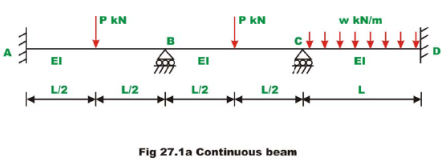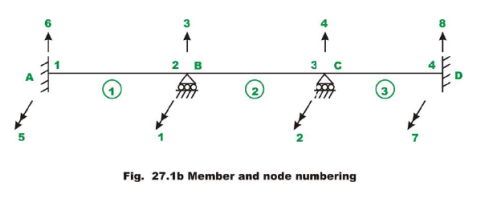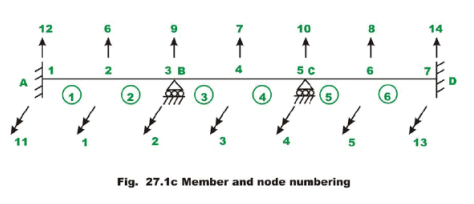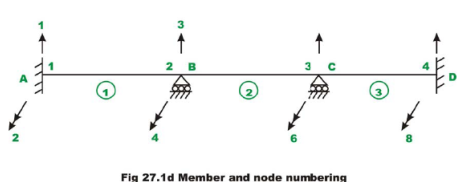In the above figures, single headed arrows are used to indicate translational and double headed arrows are used to indicate rotational degrees of freedom.

Beam Stiffness Matrix.

Fig. 27.2 shows a prismatic beam of a constant cross section that is fully restrained at ends in local orthogonal co-ordinate system x'y'z' . The beam ends are denoted by nodes j and k . The x' axis coincides with the centroidal axis of the member with the positive sense being defined from j to k. Let L be the length of the member, A area of cross section of the member and Izz  is the moment of inertia about z' 'axis.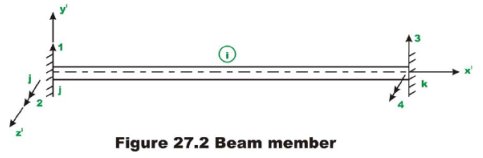Two degrees of freedom (one translation and one rotation) are considered at each end of the member. Hence, there are four possible degrees of freedom for this member and hence the resulting stiffness matrix is of the order . In this method counterclockwise moments and counterclockwise rotations are taken as positive. The positive sense of the translation and rotation are also shown in the figure. Displacements are considered as positive in the direction of the co- ordinate axis. The elements of the stiffness matrix indicate the forces exerted on the member by the restraints at the ends of the member when unit displacements are imposed at each end of the member. Let us calculate the forces developed in the above beam member when unit displacement is imposed along each degree of freedom holding all other displacements to zero. Now impose a unit displacement along y' axis at j end of the member while holding all other displacements to zero as shown in Fig. 27.3a. This displacement causes both shear and moment in the beam. The restraint actions are also shown in the figure. By definition they are elements of the member stiffness matrix. In particular they form the first column of element stiffness matrix. In Fig. 27.3b, the unit rotation in the positive sense is imposed at j end of the beam while holding all other displacements to zero. The restraint actions are shown in the figure. The restraint actions at ends are calculated referring to tables given in lesson …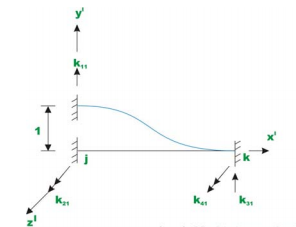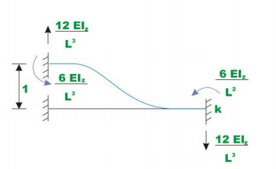(a) Unit translation along y' at end j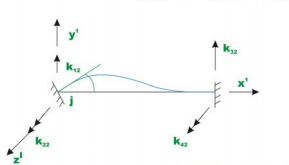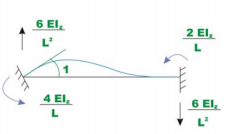(b) Unit rotation about z' at end j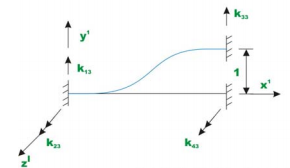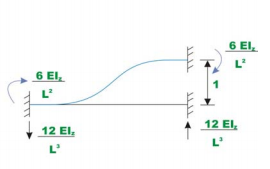(c) Unit displacement along y'at end k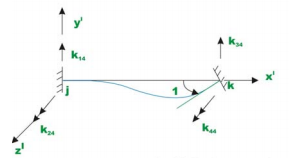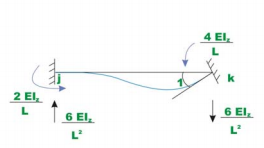(d) Unit rotation about z'at end k

Fig. 27.3 Computation of beam stiffness matrix

In Fig. 27.3c, unit displacement along y' axis at end k is imposed and corresponding restraint actions are calculated. Similarly in Fig. 27.3d, unit rotation about z' axis at end k is imposed and corresponding stiffness coefficients are calculated. Hence the member stiffness matrix for the beam member is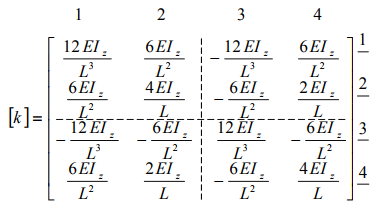(27.1)

The stiffness matrix is symmetrical. The stiffness matrix is partitioned to separate the actions associated with two ends of the member. For continuous beam problem, if the supports are unyielding, then only rotational degree of freedom shown in Fig. 27.4 is possible. In such a case the first and the third rows and columns will be deleted. The reduced stiffness matrix will be,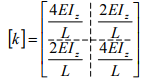(27.2)

Instead of imposing unit displacement along y' at j end of the member in Fig. 27.3a, apply a displacement u'1 along y' at j end of the member as shown in Fig. 27.5a, holding all other displacements to zero. Let the restraining forces developed be denoted by q11 , q21 , q31 and q41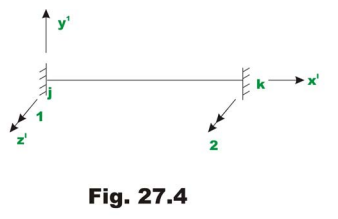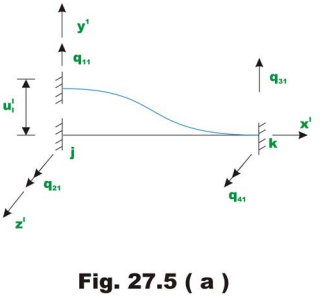The forces are equal to,

q11 = k11u'1    ;    q21 = k21u'1   ;    q31 = k31u'1    ;    q41 = k41u'1                         (27.3)

Now, give displacements u'1,u'2,u'3 and u'4 simultaneously along displacement degrees of freedom 1,2,3 and 4 respectively. Let the restraining forces developed at member ends be q1,q2,qand q4 respectively as shown in Fig. 27.5b along respective degrees of freedom. Then by the principle of superposition, the force displacement relationship can be written as,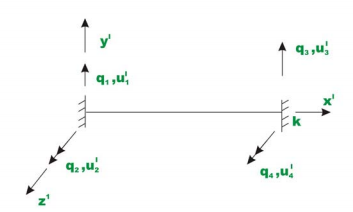Fig. 27.5 (b) Force - displacement relation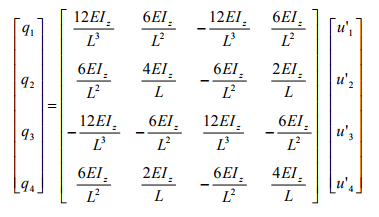(27.4)

This may also be written in compact form as,

{q} = [k] {u'}                                        (27.5)

Offer running on EduRev: Apply code STAYHOME200 to get INR 200 off on our premium plan EduRev Infinity!

## Structural Analysis

30 videos|122 docs|28 tests

,

,

,

,

,

,

,

,

,

,

,

,

,

,

,

,

,

,

,

,

,

;